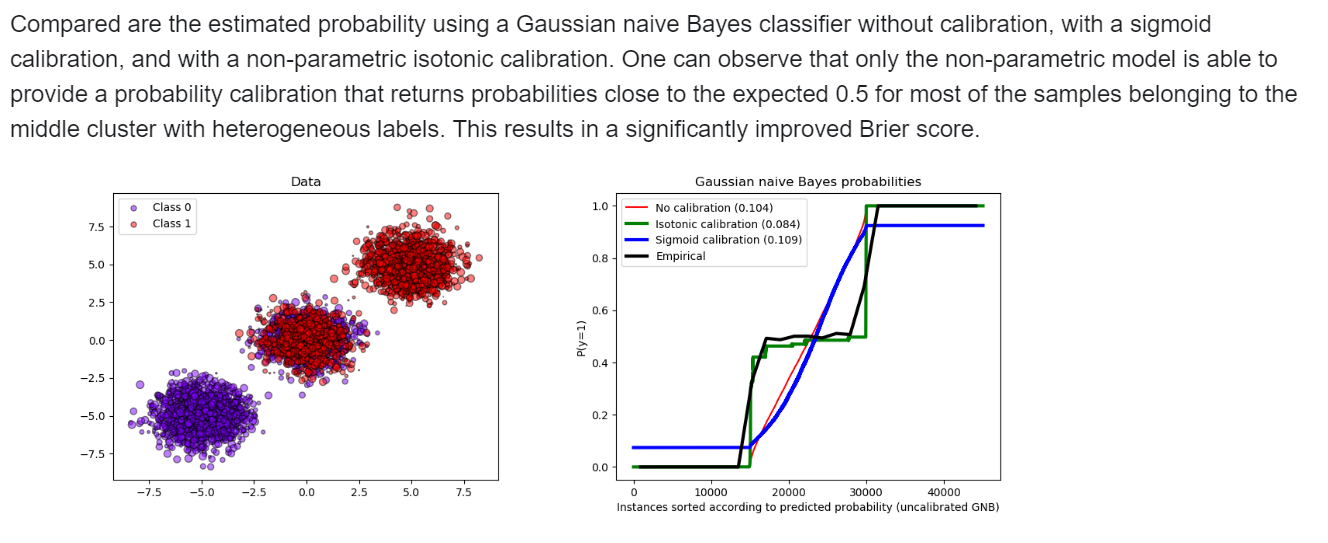Comment on page

# Calibration

## Classic Model Calibration

1. 1.
How do we do isotonic and sigmoid calibration - read this, then this, how to use in sklearn
2. 3.
TODO: how to calibrate a DNN (except sklearn wrapper for keras)
3. 4.
Allows us to use the probability as confidence. I.e, Well calibrated classifiers are probabilistic classifiers for which the output of the predict_proba method can be directly interpreted as a confidence level
4. 6.
The Brier score is a proper score function that measures the accuracy of probabilistic predictions.
5. 7.
Sk learn example
6. 8.
‘calibrated classifier cv in sklearn - The method to use for calibration. Can be ‘sigmoid’ which corresponds to Platt’s method or ‘isotonic’ which is a non-parametric approach. It is not advised to use isotonic calibration with too few calibration samples (<<1000) since it tends to overfit. Use sigmoids (Platt’s calibration) in this case. However, not all classifiers provide well-calibrated probabilities, some being over-confident while others being under-confident. Thus, a separate calibration of predicted probabilities is often desirable as a postprocessing. This example illustrates two different methods for this calibration and evaluates the quality of the returned probabilities using Brier’s score
7. 9.
Example 1 - binary class below, 2 - 3 class moving prob vectors to a well defined location, 3 - comparison of non calibrated models, only logreg is calibrated naturallyy_pred = clf.predict(X_test) if hasattr(clf, "predict_proba"): prob_pos = clf.predict_proba(X_test)[:, 1] else: # use decision function prob_pos = clf.decision_function(X_test) prob_pos = \ (prob_pos - prob_pos.min()) / (prob_pos.max() - prob_pos.min())

## Neural Net Calibration

### Temperature

1. 1.
(great) Softmax temperature by Harshit
2. 4.
short explanation by Majid
3. 6.
Calibration can also come in a different flavor, you want to make your algorithm certain, one trick is to use dropout layers when inferring/predicting/classifying, do it 100 times and average the results in some capacity , see this chapter on BNN
How Can We Know When Language Models Know? This paper is about calibration. “Recent works have shown that language models (LM) capture different types of knowledge regarding facts or common sense. However, because no model is perfect, they still fail to provide appropriate answers in many cases. In this paper, we ask the question “how can we know when language models know, with confidence, the answer to a particular query?” We examine this question from the point of view of calibration, the property of a probabilistic model’s predicted probabilities actually being well correlated with the probability of correctness. We first examine a state-ofthe-art generative QA model, T5, and examine whether its probabilities are well calibrated, finding the answer is a relatively emphatic no. We then examine methods to calibrate such models to make their confidence scores correlate better with the likelihood of correctness through fine-tuning, post-hoc probability modification, or adjustment of the predicted outputs or inputs. Experiments on a diverse range of datasets demonstrate the effectiveness of our methods. We also perform analysis to study the strengths and limitations of these methods, shedding light on further improvements that may be made in methods for calibrating LMs.”